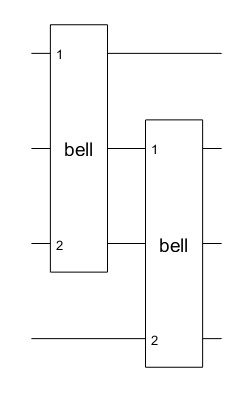# compositeGate

Construct composite gate for quantum computing

Since R2023a

Installation Required: This functionality requires MATLAB Support Package for Quantum Computing.

## Syntax

``cg = compositeGate(circuit,targetQubits)``
``cg = compositeGate(gates,targetQubits)``
``cg = compositeGate(___,Name=name)``

## Description

example

````cg = compositeGate(circuit,targetQubits)` constructs a composite gate from an inner quantum circuit and returns a `quantum.gate.CompositeGate` object. The specified target qubits map each qubit of the inner circuit to a qubit of the outer circuit containing the composite gate.The length of the `targetQubits` vector must be equal to the number of qubits in `circuit`. The `Name` property of `circuit` is copied to the `Name` property of `cg`.```

example

````cg = compositeGate(gates,targetQubits)` constructs a composite gate from an array of inner gates. The specified target qubits map each each qubit that the gates act on to a qubit of the outer circuit containing the composite gate.The length of the `targetQubits` vector must be greater than or equal to the largest qubit index in `gates`.```

example

````cg = compositeGate(___,Name=name)` sets the `Name` property of `cg` to `name`. You can use this option in addition to any of the input argument combinations in previous syntaxes.```

## Examples

collapse all

Create a quantum circuit that consists of Hadamard and controlled NOT gates to entangle two qubits. Name the circuit as `"bell"`.

```innerGates = [hGate(1); cxGate(1,2)]; innerCircuit = quantumCircuit(innerGates,Name="bell")```
```innerCircuit = quantumCircuit with properties: NumQubits: 2 Gates: [2×1 quantum.gate.SimpleGate] Name: "bell"```

Create an outer circuit that consists of two composite gates constructed from this inner `"bell"` circuit. The first composite gate acts on qubits 1 and 3 of the outer circuit containing this gate. The second composite gate acts on qubits 2 and 4 of the outer circuit containing this gate. The qubits of the inner circuit (qubits 1 and 2) are mapped to the qubits of the outer circuit (qubits 1 and 3 for the first composite gate, and qubits 2 and 4 for the second composite gate).

```outerGates = [compositeGate(innerCircuit,[1 3]) compositeGate(innerCircuit,[2 4])]; outerCircuit = quantumCircuit(outerGates)```
```outerCircuit = quantumCircuit with properties: NumQubits: 4 Gates: [2×1 quantum.gate.CompositeGate] Name: ""```

Plot the outer circuit.

`plot(outerCircuit)`In a circuit diagram, each solid horizontal line represents a qubit. The top line is a qubit with index 1 and the remaining lines from top to bottom are labeled sequentially. In this example, the plotted outer circuit consists of four qubits with indices 1, 2, 3, and 4. The plot shows that qubits 1 and 3 of the outer circuit are mapped to qubits 1 and 2 of the inner circuit of the first composite gate, and qubits 2 and 4 of the outer circuit are mapped to qubits 1 and 2 of the inner circuit of the second composite gate.

Click one of the composite gate blocks in the plot. A new figure showing the internal gates of the composite gate appears.Create an array of inner gates that consists of a Pauli X gate, a Hadamard gate, and a swap gate.

`gates = [xGate(1); hGate(2); swapGate(1,2)]`
```gates = 3×1 SimpleGate array with gates: Id Gate Control Target 1 x 1 2 h 2 3 swap [1,2] ```

Create two composite gates from the array of inner gates. The first composite gate acts on qubits 1 and 4 of the outer circuit containing this gate. The second composite gate acts on qubits 2 and 3 of the outer circuit containing this gate.

```cg1 = compositeGate(gates,[1 4],Name="custom"); cg2 = compositeGate(gates,[2 3],Name="custom"); ```

Create a quantum circuit that consists of these two composite gates.

`circuit = quantumCircuit([cg1; cg2])`
```circuit = quantumCircuit with properties: NumQubits: 4 Gates: [2×1 quantum.gate.CompositeGate] Name: ""```

Plot the circuit.

`plot(circuit)`The plotted circuit consists of four qubits with indices 1, 2, 3, and 4. The plot shows that qubits 1 and 4 of the circuit are mapped to qubits 1 and 2 of the inner gates of the first composite gate, and qubits 2 and 3 of the circuit are mapped to qubits 1 and 2 of the inner gates of the second composite gate.

## Input Arguments

collapse all

Inner circuit to construct the composite gate, specified as a `quantumCircuit` object.

Inner gates to construct the composite gate, specified as a vector containing all the inner gates. The elements of this vector are of type `SimpleGate` or `CompositeGate`.

Target qubits of the outer circuit, specified as a positive integer vector of qubit indices. The `targetQubits` vector maps each qubit of the inner circuit to a qubit of the outer circuit containing the composite gate. This vector must contain unique qubit indices.

Name of the composite gate, specified as a string scalar or character vector. The `name` input must start with a letter, followed by letters, digits, or underscores (with no white space). This name is used in the plot of the composite gate and the function name in the generated QASM code.

If you construct a composite gate from an existing `quantumCircuit` object and you do not specify this `name` input, then the `Name` property of the circuit is copied to the `Name` property of the composite gate.

## Version History

Introduced in R2023a